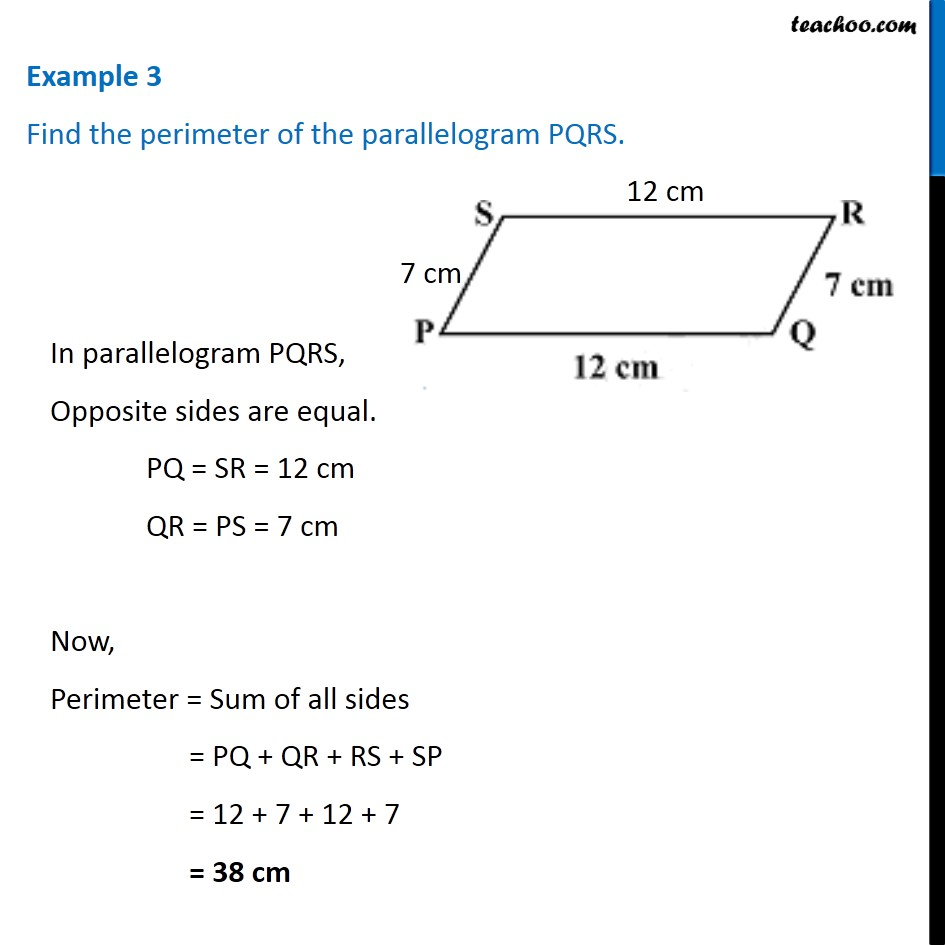Examples

Chapter 3 Class 8 Understanding Quadrilaterals
Serial order wiseLearn in your speed, with individual attention - Teachoo Maths 1-on-1 Class

### Transcript

Example 3 Find the perimeter of the parallelogram PQRS. In parallelogram PQRS, Opposite sides are equal. PQ = SR = 12 cm QR = PS = 7 cm Now, Perimeter = Sum of all sides = PQ + QR + RS + SP = 12 + 7 + 12 + 7 = 38 cm